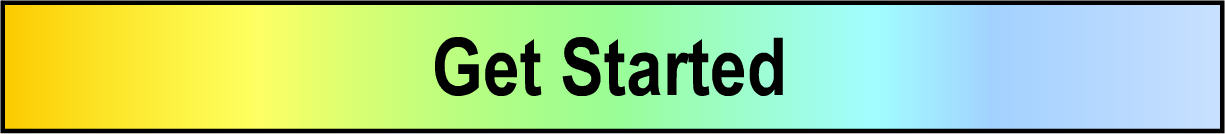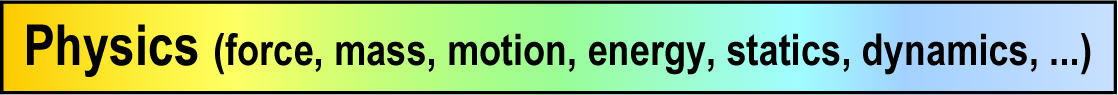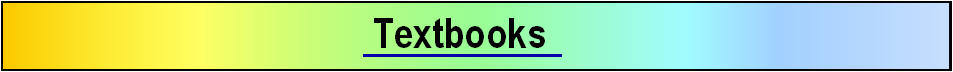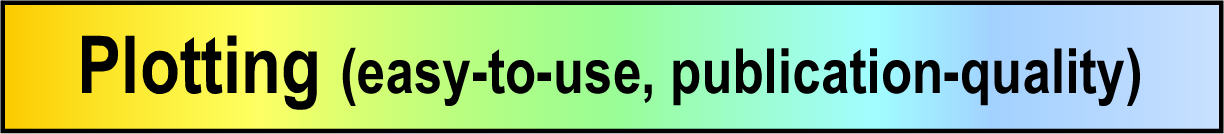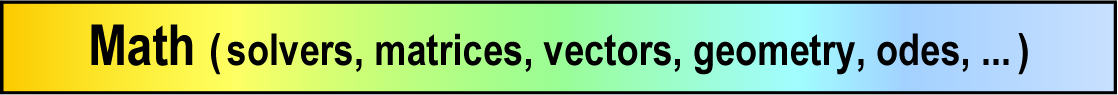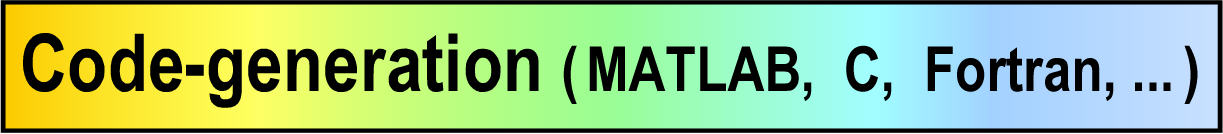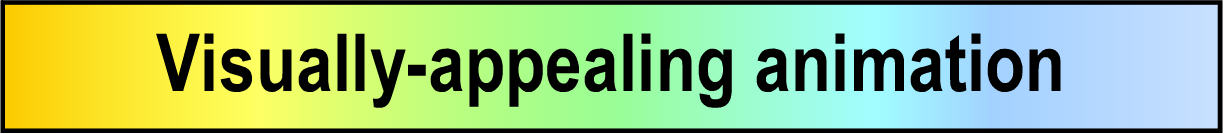MotionGenesis™ symbolic and numeric math Geometry, vectors, tensors, kinematics, ... Also linear algebra, complex numbers, ODEs, ... (writes MATLAB® .m files)

• Automatically simplifies scalars, matrices, vectors, ...
• Symbolic differentiation (partial and ordinary derivatives).
• Solves sets of linear and nonlinear differential equations (ODEs).
• Solves linear algebraic equations (symbolic and numeric matrix algebra).
• Solves sets of nonlinear algebraic equations.
• Evaluates expressions with real and complex numbers and/or symbolic expressions.
• Recursively evaluates scalar, vector, and matrix expressions (symbolically or numerically).
• Extensive unit conversions with updated NIST conversion factors
• Factors a linear or quadratic expressions or expands parentheses.
• ImaginaryNumber names sqrt(-1) for reporting results of complex calculations.
• Finds roots of polynomials (real and complex solutions) -- e.g., quadratic equations.
• Performs multi-variable Taylor series expansions.
• Automatically introduces new symbols for highly-efficient calculations
• Large library of standard built-in functions (or create your own external functions)
 ``` abs exp log log10 Factorial Squared sqrt Spline cos sin tan cosh sinh tanh acos asin atan atan2 ceil floor int round sign max min cosDegrees sinDegrees tanDegrees acosDegrees ... atan2Degrees ```Matrices, linear algebra, and eigenanalysis
• Adds, multiplies, and inverts matrices; computes determinants, eigenvalues, and eigenvectors.
• Extracts elements, rows, columns, and ranges of rows and columns from matrices.
• Creates matrix representations of vectors and dyadics, and vice-versa.
• Use matrices to solve linear and nonlinear sets of algebraic equations.
• Use matrices to solve sets of differential equations (linear/nonlinear).
• Create and use your own external matrix commands or use built-in commands, e.g.,
 ```GetColumns GetDeterminant GetDiagonalMatrix GetEigen GetElement GetIdentityMatrix GetInverse GetRows GetSVD GetTrace GetTranspose GetZeroMatrix Matrix Convolve ```

Built-in vectors, geometry, physics, and code-generation